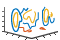# contourslice

•## 语法

``contourslice(X,Y,Z,V,xslice,yslice,zslice) ``
``contourslice(V,xslice,yslice,zslice)``
``contourslice(___,num) ``
``contourslice(___,lvls) ``
``contourslice(___,method) ``
``contourslice(ax,___)``
``s = contourslice(___)``

## 说明

````contourslice(X,Y,Z,V,xslice,yslice,zslice) ` 在三维体数据 `V` 的切片中绘制等高线，其中 `V` 决定等高线的颜色。指定 `X`、`Y` 和 `Z` 作为坐标数据。使用以下形式之一指定 `xslice`、`yslice` 和 `zslice` 作为切片位置： 要绘制一个或多个与特定轴正交的切片平面，请将切片参数指定为标量或向量。要沿曲面绘制单个切片，请将所有切片参数指定为定义曲面的矩阵。 ```
``contourslice(V,xslice,yslice,zslice)` 使用 `V` 的默认坐标数据。`V` 中每个元素的 (x,y,z) 位置分别基于列、行和页面索引。`

``contourslice(___,num) ` 指定每个切片要绘制的等高线数量。此选项可与先前语法中的任何输入参数一起使用。`
``contourslice(___,lvls) ` 指定每个切片内要绘制等高线的位置处的值。`
``contourslice(___,method) ` 指定插值方法，其中 `method` 可以是 `'linear'`、`'cubic'` 或 `'nearest'`。`
``contourslice(ax,___)` 在指定坐标区而不是当前坐标区 (`gca`) 中绘图。`
``s = contourslice(___)` 返回创建的 `Patch` 对象。`

## 示例

```[X,Y,Z,V] = flow; zslice = 0; contourslice(X,Y,Z,V,[],[],zslice) grid on``````[X,Y,Z] = meshgrid(-2:.2:2); V = X.*exp(-X.^2-Y.^2-Z.^2); xslice = [-1.2,0.8,2]; yslice = []; zslice = []; contourslice(X,Y,Z,V,xslice,yslice,zslice) view(3) grid on```x 轴上 `-1.2``0.8``2` 值处与轴正交的三个切片平面中绘制等高线。在值 `-0.2``0.4` 之间，以 `0.01` 为间距绘制三维体数据的等高线。添加颜色栏以查看数据值如何映射到等高线颜色。此外，将坐标区视图更改为三维视图并添加网格线。

```[X,Y,Z] = meshgrid(-2:.2:2); V = X.*exp(-X.^2-Y.^2-Z.^2); xslice = [-1.2,0.8,2]; lvls = -0.2:0.01:0.4; contourslice(X,Y,Z,V,xslice,[],[],lvls) colorbar view(3) grid on``````[X,Y,Z] = meshgrid(-5:0.2:5); V = X.*exp(-X.^2-Y.^2-Z.^2); [xsurf,ysurf] = meshgrid(-2:0.2:2); zsurf = xsurf.^2-ysurf.^2; contourslice(X,Y,Z,V,xsurf,ysurf,zsurf,20) view(3) grid on```## 输入参数

x 轴坐标数据，指定为向量或与 `V` 大小相同的三维数组。如果指定三维数组，它必须单调正交分布，就好像是由 `meshgrid` 函数生成的一样。

y 轴坐标数据，指定为向量或与 `V` 大小相同的三维数组。如果指定三维数组，它必须单调正交分布，就好像是由 `meshgrid` 函数生成的一样。

z 轴坐标数据，指定为向量或与 `V` 大小相同的三维数组。如果指定三维数组，它必须单调正交分布，就好像是由 `meshgrid` 函数生成的一样。

x 轴切片值，以下列形式之一指定：

• 标量 - 在指定位置绘制一个与 x 轴正交的切片平面。

• 向量 - 在指定位置绘制多个与 x 轴正交的切片平面。

• `[]` - 不绘制任何切片平面。

• 矩阵 - 沿曲面而不是平面绘制切片。如果使用此选项，`yslice``zslice` 也必须是具有相同大小的矩阵。

y 轴切片值，以下列形式之一指定：

• 标量 - 在指定位置绘制一个与 y 轴正交的切片平面。

• 向量 - 在指定位置绘制多个与 y 轴正交的切片平面。

• `[]` - 不绘制任何切片平面。

• 矩阵 - 沿曲面而不是平面绘制切片。如果使用此选项，`xslice``zslice` 也必须是具有相同大小的矩阵。

z 轴切片值，以下列形式之一指定：

• 标量 - 在指定位置绘制一个与 z 轴正交的切片平面。

• 向量 - 在指定位置绘制多个与 z 轴正交的切片平面。

• `[]` - 不绘制任何切片平面。

• 矩阵 - 沿曲面而不是平面绘制切片。如果使用此选项，`xslice``yslice` 也必须是具有相同大小的矩阵。

• `'linear'` - 在每个维度的相邻网格点进行线性插值。此值是切片为曲面时的默认值。

• `'cubic'` - 在每个维度的相邻网格点进行三次插值。

• `'nearest'` - 最近的网格点值。此值是切片为平面时的默认值。

## 算法

`contourslice` 函数不会在绘图之前清除现有图。